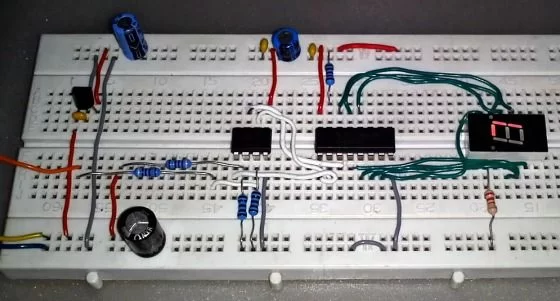# Digital logic probe for troubleshooting TTL and CMOS circuits using PIC12F683

A logic probe is considered as a stethoscope for engineers and technicians for debugging digital logic circuits that consists of logic gates, memories, registers, etc. A digital multimeter (DVM) can also be used for such analytical purposes but it gives you the numeric value of the voltage at a point instead of the logic state. Depending on whether the circuit is based on TTL or CMOS components, the voltage levels for logic 0 and 1 could be different for each family. DVM users, thus, have to calculate logic levels from the measured voltages, which consumes time and delays the troubleshooting procedure. A logic probe, on the other hand, does all these functions automatically and shows meaningful logic states at test points. In this project, we will discuss about making a digital logic probe that is applicable to both TTL and CMOS circuits and uses minimal components.Theory

There are mainly two logic families used in digital circuits: TTL and CMOS. While TTL operates at +5V, the power supply for CMOS circuits could range from +3V to 15V. According to the standard TTL convention, any voltage less than 0.8V is defined as logic low while any voltage above 2.2 is defined as logic high and anything in between these extremes is defined as intermediate state. In case of CMOS circuits, any voltage less than 1/3 of VDD is defined as logic low while any voltage above 2/3 of VDD is defined as logic high. Any voltage between these values is defined as intermediate or transitional state. This logic probe works for both TTL and CMOS circuits powered from +5V to 15V.

This logic probe consists of a 78L05 voltage regulator, a Microchip PIC12F683 microcontroller, a CD4094BE CMOS shift register and a tiny seven segment display. Since the operating voltage range of this logic probe is between 5 to 15V, the role of the 78L05 voltage regulator is to ensure that the microcontroller and the rest of the circuit are always powered with a stable 5V source. There are two voltage divider networks, each made from a 9.1K  and a 1K Ohms (1% tolerance) resistors. The outputs of the two networks go to the ADC channels AN0 and AN1. The AN0 channel reads the logic level voltage of the test pin whereas the AN1 channel is used to measure the supply voltage of the test circuit. The information of the supply voltage is necessary to identify a CMOS circuit powered with > 5V. The GP2, GP4, and GP5 pins of PIC12F683 drives the CD4094 shift register. The shift register acts as an I/O expander in this application and its outputs are connected to a seven segment display.

Software

The software for this project is written in MikroC PRO for PIC version 5.30. Only the ADC built-in library is used. The rest of the code is written without any library aid. The code starts with the definitions of pin names and common variable declarations. Apart from the main function which does all the tasks, there are six functions in the code. The setup() function sets directions of I/O pins and initiates the built-in ADC for data acquisition. The test_display() function tests the LEDs of the seven segment display connected to the shift register. The way this is done with the shift register will be discussed later in this text. The feature that makes this logic probe universal and unique is its ability to determine the supply voltage level. In the function test_supply(), the power source is tested.